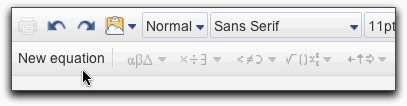14Aug/13Off

# Mathematical equations

### Insert an equation

You can easily insert mathematical equations into your documents and collaborate on a single equation at once with multiple people. Follow these steps to add an equation to your document:

1. Click the Insert drop-down menu and select Equation.
2. Select the mathematical symbol you want to add from one of these menus:
• Greek letters
• General operators
• Comparison and inclusion operators
• Operators with variables
• Arrows
1. Click the symbol you'd like to include, and add numbers or substitute variables in the box. If you delete the box by mistake, simply click New equation, and then select an option from one of the menus.If you'd like to edit the equation afterwards, simply click the equation within the document and change it. If you don't see the equation toolbar, click View and select Show equation toolbar.